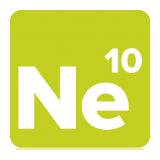Project Ne10 An open, optimized software library for the ARM architecture.
NE10_sample_matrix_multiply.c File Reference
`#include <stdio.h>`
`#include <stdlib.h>`
`#include "NE10.h"`

Go to the source code of this file.

## Macros

#define MATRICES   3

## Functions

int matrix_multiply_sample_main (void)

## Macro Definition Documentation

 #define MATRICES   3
Examples:
NE10_sample_matrix_multiply.c.

Definition at line 32 of file NE10_sample_matrix_multiply.c.

## Function Documentation

 int matrix_multiply_sample_main ( void )
Examples:
NE10_sample_matrix_multiply.c.

Definition at line 41 of file NE10_sample_matrix_multiply.c.